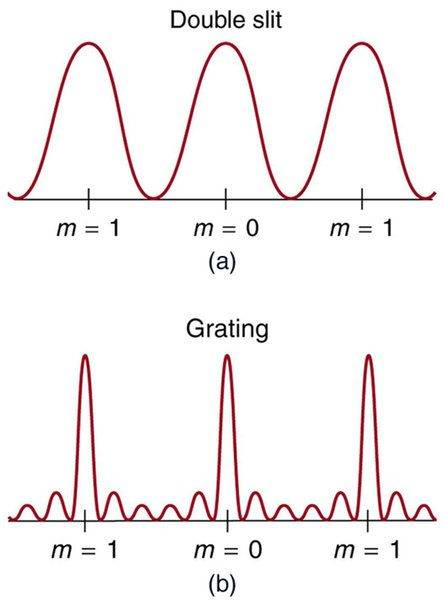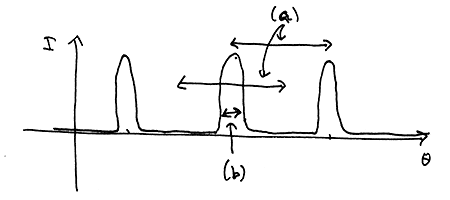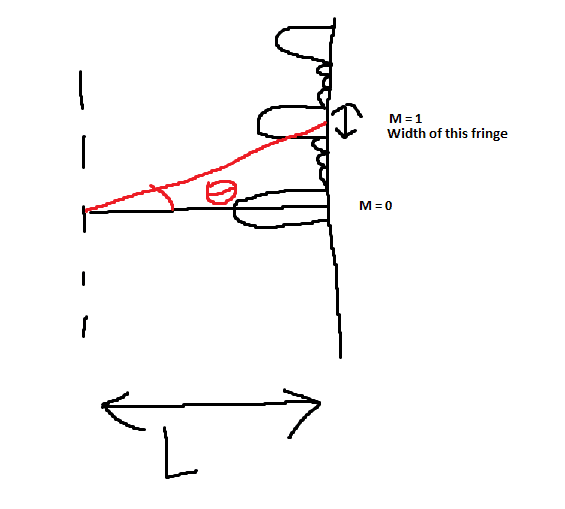# Single Slit and Diffraction Grating Formulas

• Abu

#### Abu

Hi everyone. I am having some difficulty understanding the formulas of single slit diffraction and diffraction gratings. This is partly because the formulas that I have been given in class do not reflect what I have found online entirely.

As we know, the formula for single slit destructive interference is:
dsinθ = mλ where m = 1,2,3 etc. because there is not destructive interference at the zeroth order.

However, in class we were also told that there is a formula for single slit constructive interference as well:
dsinθ = (m+1/2)λ where m = 0, 1, 2, 3 etc. When I look online and look into other physics books, I do not find this formula for single slit constructive interference. In fact, I only find the formula related to destructive.

My next confusion is with the formulas of diffraction grating
The formula I learned for constructive interference in this case is:
dsinθ = mλ which is perfectly fine.

But in class, I was also given a formula for destructive interference:
dsinθ = (m+1/2)λ
I learned from previous discussions with the great people on these forums that dsinθ = (m+1/2)λ is not applicable for destructive interference on diffraction grating. Following up on this, the interference pattern for diffraction grating, compared to double slit, is:As we can see, for double slit, the area in which destructive interference entirely occurs is in the middle of two bright orders. For that reason, dsinθ = (m+1/2)λ makes sense to me. But clearly that is not the case for diffraction grating, because there are numerous points between the bright orders where complete destructive interference occurs.
EDIT: I found a site (http://www.pa.uky.edu/~cao/NOTES-SPRING06/ch24_lecture-2-S06.pdf) that said dsinθ = (m+1/2)λ does apply for destructive interference in diffraction grating. This is beginning to add more confusion..

Is anyone able to help me clear this confusion or misunderstanding. Are the formulas that I mentioned I am confused about truly not applicable to the type of diffraction described?

Thank you.

Side note: I just watched a couple more resources and I found that dsinθ = (m+1/2)λ for constructive interference of single slit diffraction is an approximate. I think that is good enough to clear that confusion, but my confusion of the diffraction grating destructive interference still resides.

#### Attachments

Last edited by a moderator:

However, in class we were also told that there is a formula for single slit constructive interference as well:
dsinθ = (m+1/2)λ

This gives the locations of the maxima only approximately. In single-slit diffraction, the maxima are not exactly halfway between the minima. This is related to the fact that the heights of the maxima decrease rapidly as we go outward from the center.

See if you can find the formula that gives the shape of the single-slit diffraction pattern, i.e. intensity versus position. If you know calculus, you can find the locations of the maxima by the usual technique of finding the derivative of that function and setting it to zero.

My next confusion is with the formulas of diffraction grating
The formula I learned for constructive interference in this case is:
dsinθ = mλ which is perfectly fine.

This gives the locations of the principal maxima: the tallest peaks in the graph that you showed.

But in class, I was also given a formula for destructive interference:
dsinθ = (m+1/2)λ
I learned from previous discussions with the great people on these forums that dsinθ = (m+1/2)λ is not applicable for destructive interference on diffraction grating. Following up on this, the interference pattern for diffraction grating, compared to double slit, is:
View attachment 216406

That is the pattern for multiple slit interference, where the slits are very narrow. Specifically, this is for five slits. In general, if there are N slits, then between successive principal maxima there are N-1 secondary maxima. (See here for examples: http://hyperphysics.phy-astr.gsu.edu/hbase/phyopt/mulslidi.html) As N increases, there are more secondary maxima, spaced closer together. Also, their "heights" decrease with increasing N. In a decent diffraction grating, N is rather large, which makes the secondary maxima very faint, very closely spaced, and difficult to observe. This is probably why the notes in your link below simply ignore the secondary maxima.

EDIT: I found a site (http://www.pa.uky.edu/~cao/NOTES-SPRING06/ch24_lecture-2-S06.pdf) that said dsinθ = (m+1/2)λ does apply for destructive interference in diffraction grating.

I would definitely not use that formula with a diffraction grating, because there are actually many minima between the primary maxima; and even if you choose to ignore the secondary maxima, you now have in effect a broad region of effectively zero intensity, between the primary maxima; not simply a single point of minimum intensity. Note the graph on the right side of the fifth row in those notes.

•Abu
This gives the locations of the maxima only approximately. In single-slit diffraction, the maxima are not exactly halfway between the minima. This is related to the fact that the heights of the maxima decrease rapidly as we go outward from the center.

See if you can find the formula that gives the shape of the single-slit diffraction pattern, i.e. intensity versus position. If you know calculus, you can find the locations of the maxima by the usual technique of finding the derivative of that function and setting it to zero.

This gives the locations of the principal maxima: the tallest peaks in the graph that you showed.

That is the pattern for multiple slit interference, where the slits are very narrow. Specifically, this is for five slits. In general, if there are N slits, then between successive principal maxima there are N-1 secondary maxima. (See here for examples: http://hyperphysics.phy-astr.gsu.edu/hbase/phyopt/mulslidi.html) As N increases, there are more secondary maxima, spaced closer together. Also, their "heights" decrease with increasing N. In a decent diffraction grating, N is rather large, which makes the secondary maxima very faint, very closely spaced, and difficult to observe. This is probably why the notes in your link below simply ignore the secondary maxima.

I would definitely not use that formula with a diffraction grating, because there are actually many minima between the primary maxima; and even if you choose to ignore the secondary maxima, you now have in effect a broad region of effectively zero intensity, between the primary maxima; not simply a single point of minimum intensity. Note the graph on the right side of the fifth row in those notes.

Thank you so much for your response and time. I don't want to accidentally make a homework thread, but I got another question regarding the diffraction grating we just discussed.

Lets say we were finding the width of a fringe created by diffraction grating. A bright spot is surrounded by a dark spot above it and a dark spot below it. So, before seeing your response, I would attempt to use the dsinθ = (m+1/2)λ for the destructive interference to find angle theta to each dark spot, and use trigonometry to find the width of the fringe. However, we established that dsinθ = (m+1/2)λ is extremely inaccurate as there are numerous minima, not simply 1, like in the double slit. What other method would you use then, instead of that? If it is too vague, let me know if it is okay to throw in some numbers without making this too much like homework.

Once again, thank you.

Lets say we were finding the width of a fringe created by diffraction grating.
Just to make this clear, do you mean something more like (a) or (b) in the diagram below? And, what information do you have about the grating?#### Attachments

Just to make this clear, do you mean something more like (a) or (b) in the diagram below? And, what information do you have about the grating?

View attachment 216422
Sorry, I should have clarified that. It would be more like B - the actual width of the fringe itself. Let's say you knew the wavelength, what order it was (m value, let's say it is the first order) the distance between slits, and the length between the screen and the slits themselves. Essentially, you would have everything you need to solve theta for dsinθ = mλ. I would give numbers as an example but I don't know if that is permitted on this forum. The problem is that sinθ = mλ/d would give the angle to the center of the fringe, correct? In that case I don't see how it is possible without the dsinθ = (m+1/2)λ since it is not applicable to diffraction grating.The first thing I would do, I suppose, is use dsinθ = mλ to find angle theta. Then do tanθ = Opposite/L and solve for opposite. I noticed that for other problems, if they gave you a range of wavelengths for each order, you can simply find the angles to the highest and lowest wavelength and use simple trig to figure out the width. However, that is not the case here.

#### Attachments

Last edited by a moderator:
OK, the width that you're looking for is related to the resolvance or resolution of a diffraction grating.

http://hyperphysics.phy-astr.gsu.edu/hbase/phyopt/gratres.html

That page has the equations that are related to this. He measures the "width" as the actual width Δy on a screen a distance D from the slits, where y is the distance from the center of the screen. He uses the small-angle approximation sin θ ≅ tan θ = y/D.

If you want to go further with a specific example or exercise, you'd best start a new thread in one of the homework forums.

Last edited: